Skip to main content

# Initial analysis for characterizing and mitigating the pseudorange biases of BeiDou navigation satellite system

## Abstract

Pseudorange bias has become a practical obstacle in the field of high-precision global navigation satellite system (GNSS) applications, which greatly restricts the further development of high-precision applications. Unfortunately, no studies have been conducted on the pseudorange biases of the BeiDou navigation satellite system (BDS). To mitigate the effects of pseudorange biases on the BDS performance to the greatest extent possible, the origin of such BDS pseudorange biases are first thoroughly illustrated, based upon which the dependency of the biases on the receiver configurations are studied in detail. Owing to the limitations regarding the parameter re-settings for hardware receivers, software receiver technology was used to achieve the ergodicity of the receiver parameters, such as the correlator spacing and front-end bandwidth, using high-fidelity signal observations collected by a 40-m-high gain dish antenna at Haoping Observatory. Based on this, the pseudorange biases of the BDS B1I and B3I signals and their dependency on different correlator spacings and front-end bandwidths were adequately provided. Finally, herein, the suggested settings of the correlator spacing and front-end bandwidth for BDS receivers are in detail proposed for the first time. As a result, the pseudorange biases of the BDS signals will be less than 20 cm, reaching even under 10 cm, under this condition. This study will provide special attention to GNSS pseudorange biases, and will significantly promote a clear definition of the appropriate receiver parameter settings in the interface control documents of BDS and other individual satellite systems.

## Introduction

A satellite navigation signal is one of the most important factors for a global navigation satellite system (GNSS) because the internal quality of the navigation signal directly determines the performance of a GNSS service. With the extension of GNSS applications and the increasing requirements for a GNSS service refinement, there has been a growing demand for continuous improvements in the positioning, navigation, and timing (PNT) performance of a GNSS . GNSS users must rely solely on the broadcast downlink signals to obtain PNT results with high precision. Unfortunately, unexpected signal deformations may lead to differential pseudorange biases for different receivers [2,3,4]. These biases are crucial for high-precision applications, particularly for the performance and integrity of augmentation systems. In addition, pseudorange biases will have a strong impact on the precise point positioning (PPP) with an ambiguity resolution (AR) . Consequently, it is quite significant to conduct studies on the generation mechanism and characteristics of pseudorange biases in detail.

In fact, pseudorange biases were first reported during the 1990s. In Ref. , the authors indicated that satellite signals are affected by satellite-dependent pseudorange biases when estimating all electron content from GPS data. Triggered by a signal deformation of the GPS space vehicle number (SVN) 19 in 1993, which led to a significant degradation of the PNT performance when used for a differential resolution, numerous publications have mainly concentrated on the characterization of satellite signal deformations [7,8,9,10,11,12,13]. Research results have shown that receivers with different configurations, such as a different correlator spacing or different front-end bandwidth, exhibit inconsistent pseudorange biases to individual signal deformations for a GPS satellite .

Compared with GPS, there have been fewer studies conducted on the signal deformations and pseudorange biases of a BDS system. When monitoring the signal characteristics of BDS-2 and BDS experimental satellites in later 2016, it was found that there are even more serious pseudorange biases for China’s BDS system, with the largest bias being more than 1 m. However, compared with GPS, the diverse types of satellites and manufacturers, different receiver characteristics, and mismatched receiver configurations make the manifestation of pseudorange biases of BDS signals much more extreme than those of GPS or other GNSS signals.

To mitigate the pseudorange biases of GPS, the applicable values of the correlator spacing and front-end bandwidth are clearly defined in the interface control documents (ICDs) [15,16,17]. However, the ICDs for other GNSSs such as BDS and Galileo do not currently provide such a definition [18,19,20,21,22,23].

We mainly studied the origin and characteristics of the pseudorange biases of the BDS B1I and B3I civil signals, and above all, on an effective method for mitigating them. Thus, the origin of BDS pseudorange biases is analyzed in the first section. Then, based on this analysis, two affecting factors of signal deformation and receiver configuration on pseudorange biases are studied in detail. Next, based on high-fidelity signal observations collected by high-gain dish antenna and software receiver technologies, the pseudorange biases of BDS B1I and B3I signals and their dependency on different correlator spacings and different front-end bandwidths are provided. Finally, to mitigate the pseudorange biases of BDS, several effective and feasible measurements are clearly proposed based on the collected observations of BDS signals. We hope that this study will promote a clear definition of the appropriate values of the correlator spacing and bandwidth of BDS receivers, similar to those of GPS, in BDS ICDs.

## Origin of BDS pseudorange biases

Our study demonstrates that a chip shaped distortion is the root cause of pseudorange biases. However, receivers with different configurations exhibit different biases to individual signal deformations. Herein, we analyze the origin of pseudorange biases from two dimensions: the satellite and user aspects. In terms of the satellite aspect, we will introduce how the chip shapes affect the tracking biases. In addition, in terms of the user aspect, we will deduce from pseudorange observation equations how receivers respond differently to individual satellite signal distortions.

### Satellite signal deformation

Taking the BDS-2 satellite signal as an example, the shapes of the BDS signal code chips are typically rectangular, as shown in Fig. 1. Satellite-generated signals must travel a long distance before they are processed at the user’s receiver for a PNT service. Herein, we combine the entire trip as a full channel $$H_{i} (f)$$, including the on-board channel, atmospheric channel, and receiver channel. When $$i = 0$$, we assume there are no distortions throughout the entire channel. However, when $$i = 1$$, it indicates that the channel is experiencing distortion to a certain extent. If the bandwidth B is extremely wide, there will be no deformations in the signals after traveling through $$H_{0} (f)$$. By contrast, a distorted channel may result in nominal signal deformations, which will probably lead to ranging errors in a BDS receiver.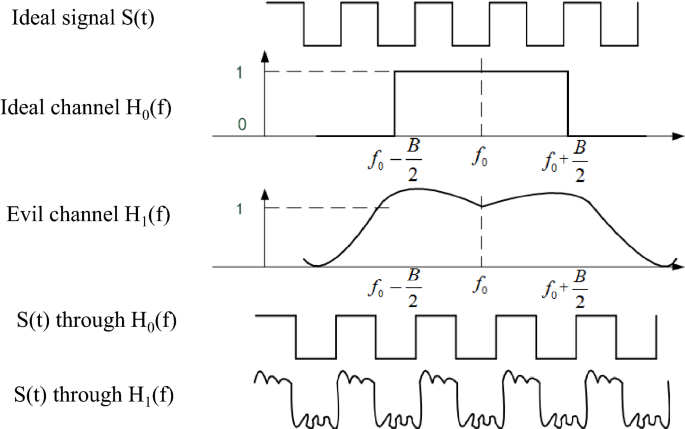Fig. 1

The correlation peak curve between a distorted signal and a locally generated ideal signal is likely deformed to a certain extent, which may lead to ranging errors and tracking biases, and will finally influence the positioning results, as shown in Fig. 2.Fig. 2

To characterize the pseudorange biases from a signal deformation occurring at a satellite, we use the variation of the early-minus-late correlator (EML) tracking biases across a range of correlator spacings to convey how the users will be affected by the severity of a signal deformation. In this case, tracking biases can be obtained from the difference between an early correlator and a late correlator. From Fig. 2, we can see that, compared with the ideal signal, the correlation peak curve of the deformed signal is distorted and asymmetric, which may lead to severe tracking biases for different receiver designs.

The tracking biases can be calculated as follows: The difference in the corresponding points of the early correlator and late correlator outputs is first calculated, and then scaled by a factor of A:

$$\varepsilon (\tau ) = \left[ {E(\tau /2) - L(\tau /2)} \right] \cdot A$$
(1)
$$A = \frac{1}{2P} \cdot \frac{1}{{f_{B} }} \cdot c$$
(2)

where τ indicates the receiver correlator spacing, or the distance between an early correlator and a late correlator, ε(τ) is the tracking error, E(τ/2) is the value of the early (E) correlator, L(τ/2) is the value of the late (L) correlator, P is the value of the prompt (P) correlator, fB denotes the frequency of the BDS PRN code, and c is the speed of light.

Figure 3 shows the tracking biases obtained from the correlation peak curve of the ideal signal and an erroneous signal, which are shown in Fig. 2. It is clear how the signal deformation influences the tracking error.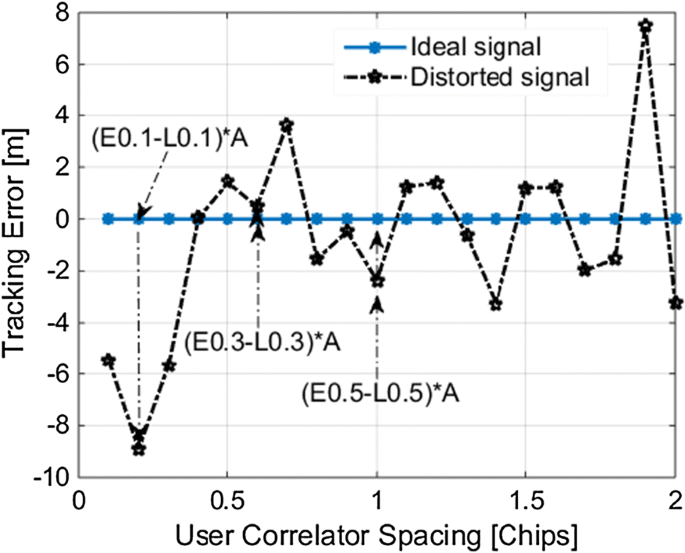Fig. 3

As with most common BDS user receivers, because the correlator spacings, filter characteristics, RF bandwidth, and discriminator types are extremely different for different receiver manufactories, different receivers may respond differently to an individual signal deformation. In the next section, we introduce how a receiver will respond differently based on a theoretical derivation.

### Receiver influence analysis

Our main concern regarding pseudorange biases is their impact on GNSS users, particularly the potential amplification that occurs when dual-frequency pseudoranges are applied to eliminate an ionospheric influence.

The common pseudorange observation equations can be expressed as follows :

$$P_{{B_{k} ,m}}^{i} = \rho_{m}^{i} + c\delta t_{m} - c\delta t^{i} + c \cdot IFB_{m} - c \cdot Tgd^{i} - iono_{m}^{i} - trop_{m}^{i} - rel_{m}^{i} - \alpha_{m}^{i} + \varepsilon_{m}^{i} + MP_{m}^{i} + SErro_{m}^{i}$$
(3)
$$P_{{B_{k} ,n}}^{i} = \rho_{n}^{i} + c\delta t_{n} - c\delta t^{i} + c \cdot IFB_{n} - c \cdot Tgd^{i} - iono_{n}^{i} - trop_{n}^{i} - rel_{n}^{i} - \alpha_{n}^{i} + \varepsilon_{n}^{i} + MP_{n}^{i} + SErro_{n}^{i}$$
(4)
$$P_{{B_{k} ,m}}^{j} = \rho_{m}^{j} + c\delta t_{m} - c\delta t^{j} + c \cdot IFB_{m} - c \cdot Tgd^{j} - iono_{m}^{j} - trop_{m}^{j} - rel_{m}^{j} - \alpha_{m}^{j} + \varepsilon_{m}^{j} + MP_{m}^{j} + SErro_{m}^{j}$$
(5)
$$P_{{B_{k} ,n}}^{j} = \rho_{n}^{j} + c\delta t_{n} - c\delta t^{j} + c \cdot IFB_{n} - c \cdot Tgd^{j} - iono_{n}^{j} - trop_{n}^{j} - rel_{n}^{j} - \alpha_{n}^{j} + \varepsilon_{n}^{j} + MP_{n}^{j} + SErro_{n}^{j}$$
(6)

where the subscripts i and j represent satellites; Bk represents the BDS-2 frequency, with B1 = 1561.098 MHz, B2 = 1207.14 MHz, and B3 = 1268.52 MHz; subscripts m and n represent hardware receivers; $$P_{{B_{k} ,m}}^{i}$$ denotes the pseudorange; $$\rho_{m}^{i}$$ represents the true range; c is the speed of light; $$\delta t_{m}$$ represents the receiver clock bias in seconds; $$\delta t^{i}$$ represents the satellite clock bias in seconds; $$IFB_{m}$$ is the inner-frequency biases in the receiver in seconds; $$Tgd^{i}$$ is the time group delay for the inner-frequency biases on the satellite in seconds; $$iono_{m}^{i}$$ is the ionosphere delay in meters; $$trop_{m}^{i}$$ is the tropospheric delay in meters; $$rel_{m}^{i}$$ represents the relativistic effect of the delay in meters; $$\alpha_{m}^{i}$$ represents the receiver channel delay in meters; $$\varepsilon_{m}^{i}$$ represents thermal noise in meters; $$MP_{m}^{i}$$ is the multipath error in meters; and $$SErro_{m}^{i}$$ denotes a signal deformation error in the pseudorange domain in meters.

For a characterization of the pseudorange bias difference between different receiver designs, herein we assume that the receivers are connected in a zero-baseline configuration to a high-gain dish antenna. Accordingly, there is no residual ground multipath from a large dish antenna. In this case, the results of O-C double difference processing between two receivers and two satellites can likely eliminate the effects of an ionosphere delay, a tropospheric delay, a receiver clock bias, a satellite clock bias, inner-frequency biases in the receiver, and inner-frequency biases on the satellite, among other factors. Thus, the pseudorange bias is obtained.

In Ref. , the authors derived in detail the signal difference and double difference among receivers in the case of common and different correlator spacings. Research results have shown that, despite the signal and double differences, the effects of a signal deformation and multiple paths cannot be eliminated if the two receiver configurations are different. However, the effects of the signal deformation and multiple paths can be eliminated when the two receiver configurations are the same.

However, if the signal distortions of all satellites are identical, or if all receiver configurations are the same, then the response of all receivers will also be identical for all satellites. As a result, there will be one shared bias for all observations. Even if the biases are different for different receiver designs, it will not have an impact on the positioning results because such an impact will be absorbed in the estimated receiver clock. However, this is not the case for the actual signals and receivers.

## Characteristics of BDS pseudorange bias

In this section, the characteristics of BDS pseudorange bias and its influence on single- and dual-frequency applications are described in detail based on the high-fidelity of the observations of the BDS B1I and B3I signals.

### BDS time-varying bias mitigation

To obtain high-precision results of the BDS pseudorange bias caused by a signal deformation, all types of large errors that may affect the results should be removed. As a result, high-fidelity measurements of the broadcast satellite signals are required. However, the measured tracking biases depend to a certain degree on the analog distortions resulting from user measurement facilities because the characteristics of the ground facilities may change over a relative long time owing to thermal changes or aging.

To analyze and remove these temporal changes, data on the BDS IGSO-5 signals were collected using a 40 m satellite dish antenna over a 20 h period from 18:00 September 8 to 14:00 September 9, 2018 local time. The dataset presented here was collected using National Instruments (NI) data acquisition (DAQ) equipment at hourly intervals. Figure 4 shows the measurement results of the pseudorange bias when varying over time.Fig. 4

We assume that the correlator spacing of the reference receiver is 1.0 B3-chip, and the correlator spacings of the user receivers are [0.1 0.6 0.8 0.9] B3-chip. From this figure, we can see that the biases are relatively constant at between 20:00 and 09:00 local time, particularly when the difference in correlator spacing between the user receiver and the reference receiver is narrow. Our study also indicates that the bias is difficult to correlate with the satellite elevation.

### Single-frequency pseudorange biases

A limited bandwidth and the ability to change the correlator spacing make hardware receivers unsuitable for delving deeply into the characteristics of pseudorange biases. Consequently, we present the detailed analysis results based on high-fidelity signal observations collected using high-gain dish antenna and software receiver technology.

Taking IGSO-6 and MEO-4 of the BDS-2 satellites as an example, Fig. 5 demonstrates the measured pseudorange biases of these two satellites with different correlator spacings and bandwidths. The results show that there are significant differences between the two satellite signal deformations, and thus the pseudorange biases of the two satellites are extremely different. In addition, the pseudorange biases vary with the correlator spacing and RF bandwidth.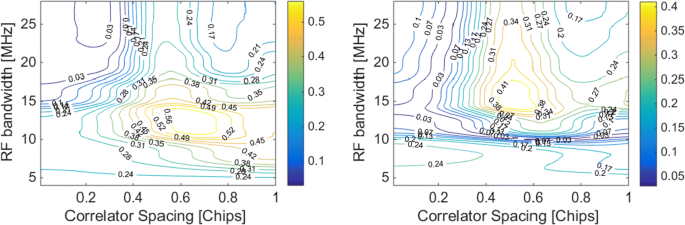Fig. 5

Figures 6, 7, 8 and 9 show the dependency of the estimated pseudorange biases of the B1 and B3 signals, respectively, on the correlator spacing and bandwidth for all BDS-2 satellites. It is also crucial to note that the shared effects do not affect the positioning accuracy, and thus the common effects are removed. As a result, only the satellite-to-satellite differences remain, which we are more concerned with.Fig. 6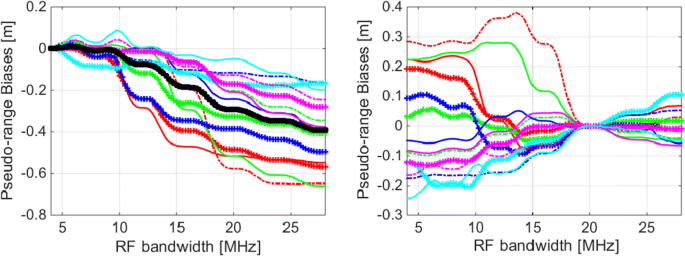Fig. 7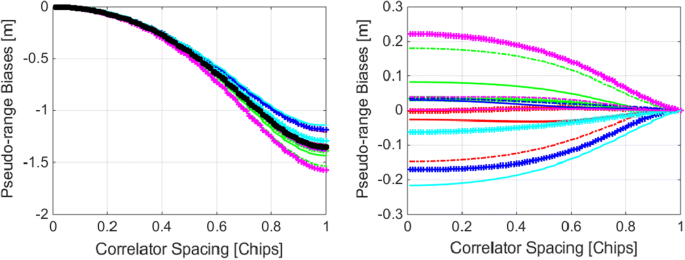Fig. 8Fig. 9

As can be seen from the plot on the left, the curves all have a similar general shape. The black line is the mean value of all curves, which will be removed from each of them. From the plot on the right, we can see that, after removing the common effects, and when a 0.2 B1-chip is considered as the reference correlator spacing, the biases between the correlator spacing range of 0.01 to 0.28 chips are then less than 10 cm, except for MEO-4, GEO-4, and GEO-1.

From Fig. 7 we can see that, when the front-end bandwidth of the reference is 20 MHz, the biases between the bandwidth range of 15–28 MHz are less than 10 cm, except for IGSO-6 and MEO-4.

Assuming that the reference receiver operates at 40 MHz with 1.0 B3-chip spacing for Figs. 8 and 9, respectively, we can obtain from the results in Fig. 8 that the biases between the correlator spacing between the 0.01 to 1.0 chips is less than 10 cm, except for IGSO-5, IGSO-1,GEO-3, GEO-1, and MEO-4. In addition, if the bandwidth of the user receiver ranges from 20 to 50 MHz, then the biases are less than 10 cm, except for those of IGSO-5, IGSO-1, GEO-3, and MEO-4, as shown in Fig. 9.

### Dual-frequency pseudorange biases

Here, we assume that receivers $$m$$ and $$n$$ can observe and receive dual-frequency signals of B1 and B3 from satellites $$i$$ and $$j$$. Then, the pseudorange observation equations of the dual-frequency combination commonly used in dual-frequency positioning can be expressed as follows :

$$P_{B1B3} \,_{mn}^{ij} = \frac{{f_{B1}^{2} }}{{f_{B1}^{2} - f_{B3}^{2} }}P_{B1} \,_{mn}^{ij} - \frac{{f_{B3}^{2} }}{{f_{B1}^{2} - f_{B3}^{2} }}P_{B3} \,_{mn}^{ij} \; = \;2.9437 \cdot P_{B1} \,_{mn}^{ij} \; - \;1.9437 \cdot P_{B3} \,_{mn}^{ij}$$
(7)

It is clear that, for the B1 and B3 dual-frequency applications, the pseudorange biases of the B1 frequency are amplified by 2.94-times, and by 1.94-times for the B3 frequency. For convenience, the linear combination of the B1 and B3 pseudoranges has the effect of amplifying the errors by a root-sum-square factor of approximately 3.5. It is obvious that, for double-frequency users, the amplification factor within the ionospheric-free pseudorange biases will be the worst when the biases of the two frequencies are negatively correlated.

Table 1 shows the calculated dual-frequency combinations of pseudorange biases from the B1 and B3 frequencies for different correlator spacings.

Here, the factor K1 in equation (7) is 2.9437, and K3 is 1.9437.

We do not provide the results of dual-frequency combinations for different RF bandwidths herein for the sake of brevity. Therefore, we simply show the following results obtained from our study:

1. 1.

Among the 14 BDS-2 satellites, PRN 14 appears to exhibit the worst dual-frequency biases for different user correlation spacings, as shown in Table 1.

2. 2.

Among the 14 BDS-2 satellites, PRN 3 appears to exhibit the worst dual-frequency biases for different user front-end bandwidths according to our research results.

3. 3.

It is clear that, for double-frequency users, the amplification factor in the ionospheric-free pseudorange biases will be the worst when the biases of the two frequencies are negatively correlated.

## Proposed mitigation methods for BDS pseudorange biases

From the above analysis, we can see that the pseudorange bias is actually a relative value, and is the difference between the pseudorange measurements of the user receiver and the reference receiver. The worst pseudorange biases occur when the reference and user receivers are mismatched.

As shown in Fig. 10, when the correlator spacing of the reference receiver is 0.5 B1-chip, and the correlator spacings of the user receivers are [0.1 0.4 0.6 1.0] B1-chip for the BDS-2 B1I signal, it is clear that reducing the differences in the correlator spacings between the user and reference receivers can significantly reduce the magnitude of the pseudorange bias.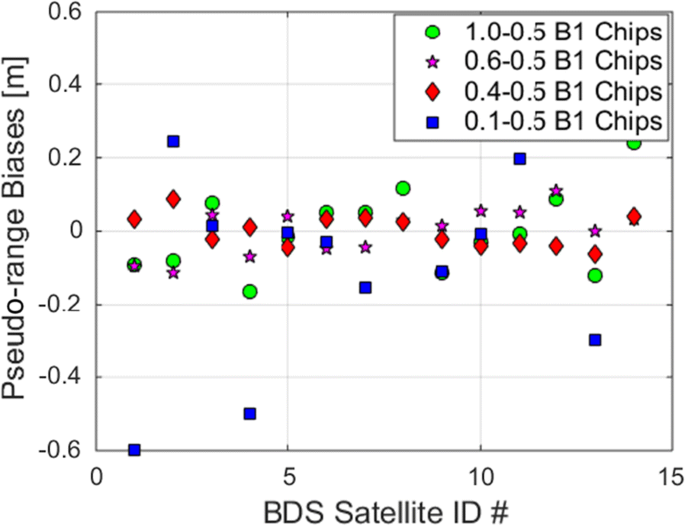Fig. 10

Consequently, to mitigate the pseudorange biases, an effective and practical way is to narrow the allowed range of user receiver configurations, such as the correlator spacing and bandwidth, more closely to the configurations of the reference receiver. Another practical way is to make sure that the configurations of all user receivers are close to each other, which does not require a match between the user receiver and the reference receiver because errors such as a signal deformation error or a multipath error can be removed through the single or double difference. In addition, a third method exists for mitigating pseudorange biases, which requires the on-board satellite signals to be constrained and modified. It is necessary that the satellite system be able to measure and modify the signal deformation with high precision. However, this method requires high-precision technology and is relatively difficult to implement. Because the first two methods are basically the same, herein we introduce in detail only the first mitigation method.

Assume that the configurations of the reference receiver for the B1 and B3 frequencies are as follows: a 0.5 B1-chip spacing and 20 MHz bandwidth for the B1 frequency, and a 1.0 B3-chip spacing and 50 MHz bandwidth for the B3 frequency. The color-filled contour plots and the proposed user/receiver correlator spacings and bandwidths for the BDS B1I and B3I signals are as shown in Figs. 11 and 12, respectively.Fig. 11Fig. 12

The proposed allowed settings of the correlator spacing and front-end bandwidth for BDS user receivers are highlighted within the red rectangular regions in each figure. Note that the largest pseudorange bias for B1I is approximately 1.54 m, and for B3I is approximately 1.88 m. However, the pseudorange biases can probably be reduced to less than 20 cm within the regions highlighted, which are the areas closest to the reference receiver configuration.

The results obtained from this study can be attributed to the requisite theory for a clear definition of the appropriate values of the user receiver configuration, which will be applied in BDS ICDs in the near future.

## Conclusions

Signal deformations and their differences among GNSS satellites are the essential triggers for an individual pseudorange bias. However, receivers with different configurations exhibit different biases to individual signal deformations. Consequently, pseudorange biases occur.

This study considered comprehensively the essential reasons and characteristics of all civil signals of BDS-2 satellites. Most importantly, we proposed for the first time several practical and effective ways to mitigate the influence of BDS pseudorange biases. The appropriate settings for a BDS receiver configuration, such as the correlator spacing and RF bandwidth, were clearly suggested. The results show that, when the configuration of the user receiver is extremely close to that of the reference receiver, the largest pseudorange bias can be reduced to less than 20 cm, or even less than 10 cm. As a result, it is suggested that all other GNSSs providers should pay more attention to their pseudorange biases, allowing us to work together to guarantee a safer and more reliable GNSS application.

## Availability of data and materials

The data supporting the findings of this study are available only from the Office of Science and Technology of the National Time Service Center; however, certain restrictions apply to the availability of these data, which were used under license for the present study, and are thus not publicly available.

## Abbreviations

BDS:

BeiDou navigation satellite system

GNSS:

Global navigation satellite system

ICD:

Interface control document

PNT:

Positioning, navigation, and timing

PPP:

Precise point positioning

AR:

Ambiguity resolution

GPS:

Global positioning system

EML:

Early-minus-late

O-C:

Observation-minus-calculation

IGSO:

Inclined geosynchronous satellite orbit

MEO:

Medium earth orbit

GEO:

Geostationary earth orbit

NI:

National instrument

DAQ:

Data acquisition

RF:

Radio frequency

## References

1. 1.

Yao, Z., & Lu, M. (2016). Principles of signal design and technologies of signal implementation for the new generation satellite navigation system. Beijing: Publishing House of Electronics Industry.

2. 2.

Wong, G., Chen, Y. H., Phelts, R. E., Walter, T., & Enge, P. (2014) Mitigation of nominal signal deformations on dual-frequency WAAS position errors. In Proceedings of the ION GNSS 2014 (pp. 3129–3147), September 8–12, Institute of Navigation, Tampa, Florida, USA.

3. 3.

Phelts, R. E., Blanch, J., Walter, T., & Enge, P. (2014) The effect of nominal signal deformation biases on ARAIM users. In Proceedings of the ION ITM 2014 (pp. 56–67), January 27–29, Institute of Navigation, San Diego, California, USA.

4. 4.

He, C., Guo, J., Lu, X., et al. (2018). A new evil waveforms evaluating method for new BDS navigation signals. GPS Solutions, 22(2), 1–13. https://doi.org/10.1007/s10291-018-0698-x.

5. 5.

Hauschild, A., & Montenbruck, O. (2015) The effect of correlator and front-end design on GNSS pseudorange biases for geodetic receivers. In Proceedings of the ION GNSS + 2015 (pp. 2835–2844), September 14-18, Institute of Navigation, Tampa, Florida.

6. 6.

Coco, D. S., Clayton, C., Scott, R. D., & James, R. C. (1991). Variability of GPS satellite differential group delay biases. IEEE Transactions on Aerospace and Electronic Systems, 27(6), 931–938.

7. 7.

Sardon, E., & Zarraoa, N. (1997). Estimation of total electron content using GPS data: How stable are the differential satellite and receiver instrumental biases. Radio Science, 32(5), 1899–1910. https://doi.org/10.1029/97RS01457.

8. 8.

Wong, G., Phelts, R. E., Walter, T., & Enge, P. (2011) Alternative characterization of analog signal deformation for GNSS-GPS satellites. In Proceedings of the ION ITM 2011 (pp. 497–507), January 24–26, Institute of Navigation, San Diego, CA.

9. 9.

Lestarquit, L., Gregoire, Y., & Thevenon, P. (2012) Characterizing the GNSS correlation function using a high gain antenna and long coherent integration—Application to signal quality monitoring. In Proceedings of the IEEE/ION PLANS 2012 (pp. 877-885), April 24–26, Myrtle Beach, SC.

10. 10.

Gunawardena, S., & Van, G. F. (2013) An empirical model for computing GPS SPS pseudorange natural biases based on high fidelity measurements from a software receiver. In Proceedings of the ION GNSS + 2013 (pp. 1341–1358), September 16–20, Institute of Navigation, Nashville, Tennessee.

11. 11.

Hauschild, A., & Montenbruck, O. (2016). A study on the dependency of GNSS pseudorange biases on correlator spacing. GPS Solutions, 20, 159–171. https://doi.org/10.1007/s10291-014-0426-0.

12. 12.

Jefferson, D. C., Michael, B. H., & Ronald, J. M. (2001). Examining the C1-P1 pseudorange bias. GPS Solutions, 4(4), 25–30.

13. 13.

Wong, G., Chen, Y., Phelts, R. E., Walter, T., & Enge, P. (2012) Measuring code-phase differences due to inter-satellite hardware differences. In Proceedings of the ION GNSS 2012 (pp. 2150–2158), September 17-21, Institute of Navigation, Nashville, Tennessee.

14. 14.

Vergara, M., Sgammini, M., Zhu, Y., Thoelert, S., & Antreich, F. (2014) Tracking error modeling in presence of satellite imperfections. In Proceedings of the ION ITM 2004 (pp. 246–253), January 27–29, Institute of Navigation.

15. 15.

IS-GPS-200-H. (2013). Global positioning systems directorate, systems engineering and integration. Interface specification, Navstar GPS space segment/navigation user interfaces (IS-GPS-200-H).

16. 16.

IS-GPS-705D. (2013). Global positioning systems directorate, systems engineering and integration. Interface specification, Navstar GPS space segment/user segment L5 interfaces (IS-GPS-705D).

17. 17.

IS-GPS-800D. (2013). Global positioning systems directorate, systems engineering and integration. Interface specification, Navstar GPS space segment/user segment L1C interface.

18. 18.

GLONASSS-ICD. (2008). Russian Institute of space device engineering. global navigation satellite system GLONASS, interface control document (Version 5.1).

19. 19.

BDS-SIS-ICD. (2013). China Satellite Navigation Office. BeiDou navigation satellite system signal in space interface control document open service signal (Version 2.0).

20. 20.

OS SIS ICD. (2010). European Union. European GNSS (Galileo) open service signal in space interface control document (OS SIS ICD), Issue 1.1.

21. 21.

OS SIS ICD (2014) European Union. European GNSS (Galileo) open service signal in space interface control document (OS SIS ICD), Issue 1.2.

22. 22.

IS-QZSS. (2014). Japan Aerospace Exploration Agency. Quasi-Zenith satellite system navigation service, interface specification for QZSS Version 1.6.

23. 23.

ISRO-IRNSS-ICD-SPS. (2014). ISRO Satellite Centre Indian Space Research Organization. Signal in space ICD for standard positioning service version 1.0.

24. 24.

He, C., Guo, J., Lu, X., Wang, X., Rao, Y., Kang, L., et al. (2018). Researches on pseudorange biases of BDS B1I signals. Journal of Electronics and Information Technology, 40(11), 2698–2704.

Download references

## Acknowledgements

The authors would like to give our sincere gratitude to the staff of the Haoping Radio Observatory of the National Time Service Center for their support and help in the radio channel calibration and GNSS signal collection. In addition, the authors would like to thank the reviewers of this paper for their constructive comments.

## Funding

This study was sponsored by the National Nature Science Foundation of China (Nos. 61501430 and 41604029) and the State Key Laboratory of Geo-information Engineering (SKLGIE2017-M-2-2)

## Author information

Authors

### Contributions

Chengyan He, Xiaochun Lu, and Ji Guo contributed to the conception of the study and contributed significantly to the analysis and manuscript writing, Wei Wang and Chengeng Su conducted the data collection and data analysis, Meng Wang conducted a data analysis and the manuscript revision, and all authors read and approved the final manuscript.

### Corresponding author

Correspondence to Chengyan He.

## Ethics declarations

### Competing interests

The authors declare that they have no competing interests.

## Additional information

### Publisher’s Note

Springer Nature remains neutral with regard to jurisdictional claims in published maps and institutional affiliations.

## Rights and permissions

Open Access This article is licensed under a Creative Commons Attribution 4.0 International License, which permits use, sharing, adaptation, distribution and reproduction in any medium or format, as long as you give appropriate credit to the original author(s) and the source, provide a link to the Creative Commons licence, and indicate if changes were made. The images or other third party material in this article are included in the article's Creative Commons licence, unless indicated otherwise in a credit line to the material. If material is not included in the article's Creative Commons licence and your intended use is not permitted by statutory regulation or exceeds the permitted use, you will need to obtain permission directly from the copyright holder. To view a copy of this licence, visit http://creativecommons.org/licenses/by/4.0/.

Reprints and Permissions